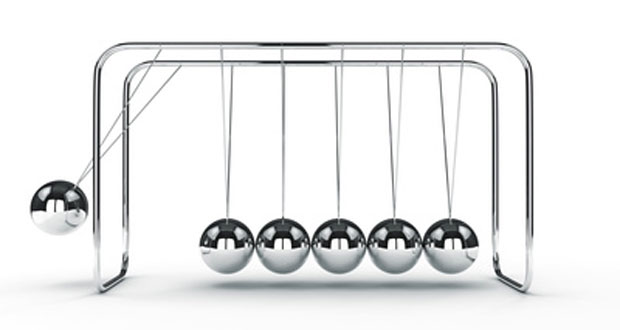# Newtons Laws of MotionSir Isaac Newton stated three laws which explain the relationship between force and movement. Having a good understanding of Newton’s laws, that can be applied to sporting situations, will allow you to gain a deeper understanding of sports technique.

## Newton’s first law of motion

“A body continues in a state of rest or uniform velocity unless acted upon by an external force.”

This is also known as the ‘law of inertia’ and means that something will either stay still or stay moving unless a force acts on it. For example, a golf ball will remain still unless a force, applied by the golf club, makes it move. Or that same golf ball will continue to move at a constant velocity unless a force acts on it to slow it down (e.g. wind resistance) or change its direction (e.g. gravity).

Questions: Can you give examples in sport where:

1. A force causes a body at rest to move?
2. A force causes a moving body to change direction?
3. A force causes a moving body to accelerate?
4. A force causes a moving body to decelerate?
5. A force causes a body to change shape?

## Newton’s second law of motion

“When a force acts on an object, the rate of change of momentum experienced by the object is proportional to the size of the force and takes place in the direction in which the force acts.”

Put more simply, this means that when the golf ball, from the example above, is struck by the golf club the rate of change of momentum (or velocity) of the ball is proportional to the size of the force acting on it by the club. A useful way of writing Newton’s second law of motion, particularly when doing calculations, is Force = mass x acceleration (or F=ma).

## Newton’s third law of motion

“For every action, there is an equal and opposite reaction.”

Or to put it exactly how Newton put it – ‘For every force that is exerted by one body on another, there is an equal and opposite force exerted by the second body on the first.’ This is sometimes referred to as the law of reaction.

For example, when a Tennis player hits a ball the racket exerts a force on the ball and the ball exerts an equal and opposite force on the racket. The racket exerts what is known as the action force and the ball exerts the reaction force which is felt by the player in the increased resistance at the time the racket strikes the ball.

If the same ball then hits the floor it exerts a force on the floor and the floor exerts an equal and opposite force on the ball.